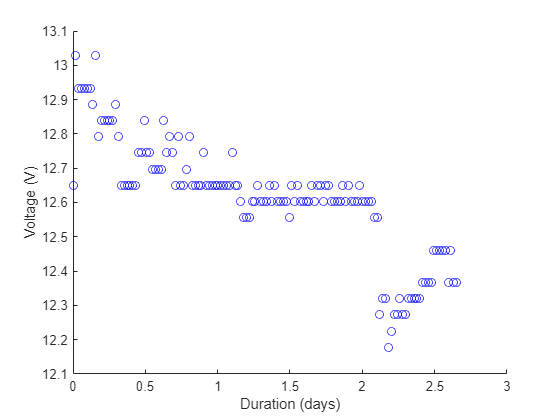Documentation

## Read ThingSpeak Data and Predict Battery Discharge Time with Linear Fit

This example shows how to read battery data from a ThingSpeak™ channel and analyze the data to determine the remaining battery life. Use a linear fit to predict the date that the battery will fail, and then write the remaining time in days to another ThingSpeak Channel. You read data for a 12 V battery connected to a microprocessor reporting its voltage to ThingSpeak every half hour. Then use regression to predict the day and time when the battery will fail.

### Read Data from ThingSpeak Channel

Start by storing channel and date information in variables, and then use `thingSpeakRead` to read the data. Channel 592680 shows the scaled measurement of voltage from a 12 V battery. Use the `DateRange` name-value pair to use a specific selection of data.

```batteryChannelID = 592680; startDate = datetime('Oct 20, 2018'); endDate = datetime('Oct 23, 2018'); batteryData = thingSpeakRead(batteryChannelID,'DateRange',[startDate endDate],'Outputformat','Timetable');```

### Convert the Data for Fitting and Plot

The channel stores raw data from the device. Convert the analog-to-digital converter (ADC) measurement to voltage using the experimentally determined conversion factor 14.6324. Then use `scatter` to generate a plot.

```myVoltage = 14.6324 * batteryData.Voltage; scatter(batteryData.Timestamps,myVoltage,'b'); ylabel('Voltage (V)'); hold on```### Fit the Data

The timetable datetime format is useful for reading and plotting. To fit the data, the datetime needs to be in numeric format. Use `datenum` to convert the timestamps into a number of days, and subtract the starting number to keep the values low. Use `polyfit` to perform linear regression on the data, and `polyval` to evaluate the fit at the existing time values. Add the fit line to the previous plot.

```battTimes = datenum(batteryData.Timestamps); battTimes= battTimes-battTimes(1); myFit=polyfit(battTimes,myVoltage,1); fitLine=polyval(myFit,battTimes); plot(batteryData.Timestamps,fitLine,'r--');```### Predict Discharge Time

The battery should not be discharged below 10.4 V. Find the number of days until the fit line will intersect with this voltage.

`endDays = (10.4-myFit(2))/myFit(1)`
```endDays = 13.1573 ```

There are just over 13 days until the battery dies.

### Write Prediction to ThingSpeak

The `thingSpeakWrite` function writes the result to a ThingSpeak channel. Return the output from `thingSpeakWrite` to ensure a successful write operation. Change the `writeChannelID` and `writeAPIKey` to write to your own channel.

```writeChannelID = 17504; writeAPIKey='23ZLGOBBU9TWHG2H'; result = thingSpeakWrite(writeChannelID,round(endDays,4),'WriteKey',writeAPIKey)```
```result = struct with fields: Field1: '13.1573' Field2: [] Field3: [] Field4: [] Field5: [] Field6: [] Field7: [] Field8: [] Latitude: [] Longitude: [] ChannelID: 17504 Created: 03-Jun-2019 15:24:43 LastEntryID: 50018 Altitude: [] ```

The result shows the successful write operation and reports the data that was written.